# Isothermal coordinates

(diff) ← Older revision | Latest revision (diff) | Newer revision → (diff)
Jump to: navigation, search

Coordinates of a two-dimensional Riemannian space in which the square of the line element has the form: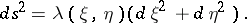Isothermal coordinates specify a conformal mapping of the two-dimensional Riemannian manifold into the Euclidean plane. Isothermal coordinates can always be introduced in a compact domain of a regular two-dimensional manifold. The Gaussian curvature can be calculated in isothermal coordinates by the formula: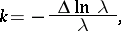whereis the Laplace operator.

Isothermal coordinates are also considered in two-dimensional pseudo-Riemannian spaces; the square of the line element then has the form: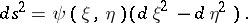Here, frequent use is made of coordinateswhich are naturally connected with isothermal coordinates and in which the square of the line element has the form:In this case the lines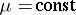and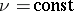are isotropic geodesics and the coordinate system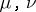is called isotropic. Isotropic coordinates are extensively used in general relativity theory.